You are here
Home > Class 11 > Binomial Theorem class 11

# Binomial Theorem class 11Chapter 8

## Binomial TheoremBinomial theorem for any positive integer n

(a+b)n = nC0 an + nC1 an-1 b + nC2 an-2 b2 + … + nCn bn

=  an + nC1 an-1 b + nC2 an-2 b2 + … +  bn

Q.  Expand= x8 + 4.x6.() + 6.x4. + 4.x2. += x8 + 12x5 + 54x2 +Q.  (97)3

(97)3  = (100-3)3

= 10033C1(100)2.3 + 3C2(100)(3)2 – 33

= 1000000 – 3.10000.3 + 3. 100.9 – 27

= 1000000 – 90000 + 2700 – 27

= 912673

### Note:

102 = 100+2

0.99 = 1- 0.01

Q.  Find (a+b)4 – (a-b)4. Hence evaluate(a+b)4 = a4 + 4C1 a3 b + 4C2 a2 b2 + 4C3 a b3 + b4

= a4 + 4a3b + 6a2b2 + 4ab3 +  b4

(a-b)4 =  a4 – 4a3b + 6a2b2 – 4ab3 +  b4

(a+b)4 – (a-b)4 = 8a3b + 8ab3

= 8ab(a2+b2)

(√3+ √2)4  – (√3- √2)4 = 8√3 √2 (√32  + √22 )

= 8√6 (3+2) = 40√6

### Note:

1.     The number of terms in the expansion of (a+b)n is n+1

2.     T1,T2,T3,…. are the terms

3.     T2 = nC1 an-1 b1

4.     T3 = nC2 an-2 b2

5.     T4 = nC3 an-3 b3

6.     Tr+1 is the general term in binomial expansion

7.   Tr+1 = nCr an-r br

Middle terms

Let m be the number of terms in a binomial expansion.

If m is odd it has only one middle term,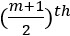term.

If m is even, it has 2 middle terms ,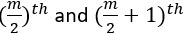term.

Q. Find the middle term in the expansion of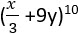T6 is the middle term

T6 = nC5 an-5 b5

= 10C5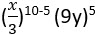= 25295y5

= 252.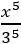.59049. y5

= 61236x5y5

Q. Consider the expansion of (x2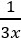)9

(i) Find the coefficient of x9

(ii) Find the term independent of x

Tr+1 = nCr an-r br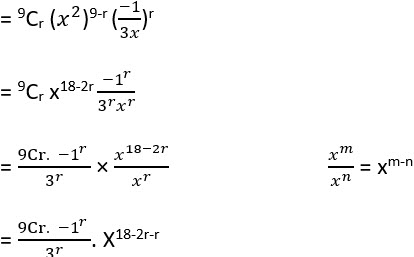18 – 3r = 9

3r = 18 -9= 9

r= 9/3 = 3

T3+1 =T4   =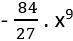The coefficient of x9 = –To find the term independent of x, put power of x in the general

term = 0

18-3r = 0

3r = 18

r = 18/3=6

T6+1T7 =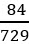Term independent of x =Q. Find a positive value of n for which the coefficient of x2 in the expansion of (1+x)n is 6

(1+x)n = 1 + nC1x + nC2x2 + … + xn

The coefficient of x2 = nC2

nC2= 6= 6

n2-n= 12

n2 – n – 12 = 0

n= 4, -3

n=4

Q. Prove that nC0 + nC1+nC2+ …. + nCn = 2n

(1+x)n = 1 + nC1x + nC2x2 + … + xn

Put x = 1

(1+1)n = 1 + nC1.1 + nC2.12 + … + 1n

2n = nC0 + nC1+nC2+ …. + nCn

### Exercise

1.    Expand ()4

2.   Find (x+1)6 + (x-1)6. Hence evaluate (√2+1)6 + (√2-1)6

3.     Find a if 17th and 18th terms of the expansion (2+a)50 are equal

4.     Find the term independent of x in the expansion of (3x2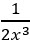)10

5.    Find the coefficient of x10 in (2x2)20

6.    Does the expansion (2x2)20 contain any term involving x9? Justify

7.    Find the fourth term from the end and middle term  in the expansion of ()9

8.     In (a+b)n, the coefficient of fourth and thirteenth terms are equal, find n

9.     In the expansion of (1+a)m+n, prove that the coefficient of am and an are equal

10.   Show that 9n+1-8n-9 is divisible by 64 where n is a positive integer using binomial theorem